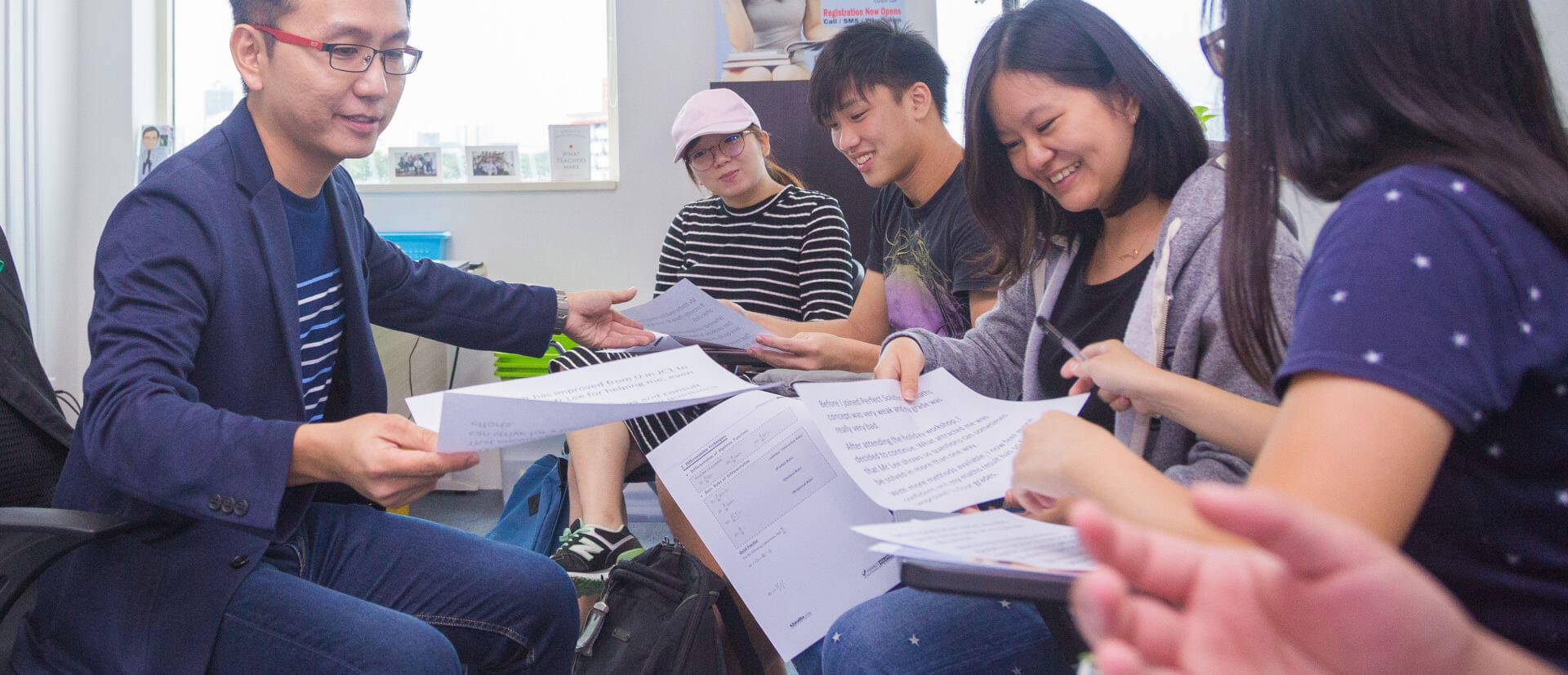# The Top 10 Math Concepts Every Singaporean Student Should Know

Singapore has long been regarded as a world leader in math education, with its students consistently ranking highly in international assessments. This success can be attributed to a strong focus on developing foundational knowledge and skills in math concepts, which are then applied to problem-solving. Here are the top 10 math concepts that every Singaporean student should know, along with an example of each concept:

Number sense: Number sense is the ability to understand the relationships between numbers and their magnitudes, and to use this understanding to perform arithmetic operations. An example of this concept is estimating the answer to a multiplication problem, such as 37 x 24, by rounding the numbers to the nearest ten and then multiplying: 40 x 20 = 800.

Fractions: Fractions are a fundamental concept in math, and are extensively taught in Singapore. An example of this concept is adding two fractions with different denominators, such as 1/3 + 1/4. To do this, students would first find a common denominator (in this case, 12), then convert each fraction to an equivalent fraction with that denominator, and finally add the numerators: 4/12 + 3/12 = 7/12.

Algebra: Algebra is the study of mathematical symbols and the rules for manipulating these symbols. An example of this concept is solving an equation, such as 2x + 5 = 13. To solve for x, students would first isolate the variable by subtracting 5 from both sides, and then divide by 2: 2x = 8, x = 4.

Geometry: Geometry is the study of shapes, sizes, positions, and properties of space. An example of this concept is finding the area of a triangle with a base of 8 units and a height of 4 units. To do this, students would use the formula for the area of a triangle: A = (base x height) / 2, and plug in the values: A = (8 x 4) / 2 = 16.

Measurement: Measurement is the process of assigning a numerical value to an attribute or property of an object or event. An example of this concept is measuring the length of a piece of string using a ruler, and recording the measurement in centimeters or millimeters.

Data analysis: Data analysis involves collecting, organizing, and interpreting data to draw conclusions and make predictions. An example of this concept is creating a bar graph to represent the number of students in a class who prefer different types of food. To do this, students would collect data from their classmates, organize it into categories (such as pizza, burgers, and sushi), and then create a graph with the number of students in each category represented by a bar.

Problem-solving: Problem-solving is a critical component of Singaporean math education. An example of this concept is solving a word problem, such as: If John has 10 apples and he gives 3 apples to Mary, how many apples does John have left? To solve this problem, students would need to read and understand the problem, identify the relevant information, and then use subtraction to find the answer: 10 – 3 = 7.

Patterns: Patterns are a recurring theme in math, and are introduced early in Singaporean math education. An example of this concept is identifying a pattern in a sequence of numbers, such as 2, 4, 6, 8, 10, and then using the pattern to predict the next number in the sequence: 12.

Probability: Probability is the study of the likelihood or chance of events occurring. An example of this concept is calculating the probability of rolling a 6 on a standard six-sided dice. In this case, the probability of rolling a 6 is 1/6, since there is only one possible outcome that results in rolling a 6 out of the six possible outcomes.

Logic and reasoning: Logic and reasoning involve using critical thinking to make deductions and draw conclusions based on given information. An example of this concept is solving a logic puzzle, such as a Sudoku puzzle, where the player must use deductive reasoning to fill in the blank cells with the correct numbers.

These 10 math concepts are just a small sample of the wide range of mathematical topics that are taught in Singaporean schools. By mastering these foundational concepts, students are equipped with the skills and knowledge necessary to tackle more complex math problems and concepts in the future.

In addition to these concepts, there are several strategies and approaches that are also emphasized in Singaporean math education. For example, the use of visual aids, such as diagrams and models, is often used to help students visualize math problems and understand abstract concepts. Another common approach is the use of problem-solving heuristics, or problem-solving strategies, which provide students with a structured method for approaching and solving problems.

Overall, Singaporean math education is characterized by a strong emphasis on foundational knowledge, problem-solving, and critical thinking skills. By mastering these key concepts and approaches, Singaporean students are able to excel in math and develop a love for the subject that will serve them well throughout their lives.